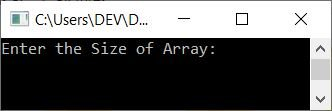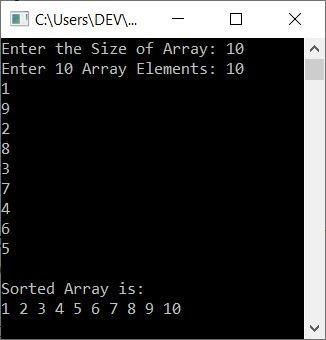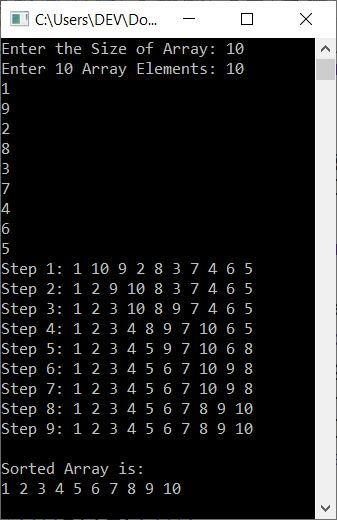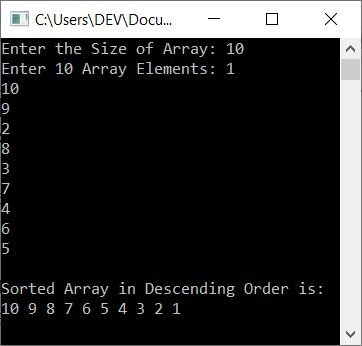# C++ Program for Selection Sort

In this article, you will learn and get code to implement selection sort in C++. Here is the list of programs on selection sorting available in this article:

But before creating these programs, if you're not aware of selection sort, refer to the selection sort algorithm and examples to get every required detail about it.

## Selection Sort in C++

To sort an array in ascending order using the selection sort technique in C++ programming, you have to ask the user to enter the size and elements of the array. Now sort the array using the selection sort technique as shown in the program given below:

Note: Selection sort works in a way that, initially, the smallest element gets selected and moved to the very first index (0th index, because indexing in arrays starts from 0), then the second smallest element gets selected and moved to the second (1st) index, and so on.

```#include<iostream>
using namespace std;
int main()
{
int tot, arr, i, j, temp, small, chk, index;
cout<<"Enter the Size of Array: ";
cin>>tot;
cout<<"Enter "<<tot<<" Array Elements: ";
for(i=0; i<tot; i++)
cin>>arr[i];
for(i=0; i<(tot-1); i++)
{
chk=0;
small = arr[i];
for(j=(i+1); j<tot; j++)
{
if(small>arr[j])
{
small = arr[j];
chk++;
index = j;
}
}
if(chk!=0)
{
temp = arr[i];
arr[i] = small;
arr[index] = temp;
}
}
cout<<"\nSorted Array is:\n";
for(i=0; i<tot; i++)
cout<<arr[i]<<" ";
cout<<endl;
return 0;
}```

This program was built and runs under the Code::Blocks IDE. Here is its sample run:Now enter the size, say 10 and then enter any 10 array elements, say 10, 1, 9, 2, 8, 3, 7, 4, 6, and 5, and press the `ENTER` key to sort the array using the selection sort technique, and then print the new sorted array as shown in the snapshot given below:The dry run of the above program with the same user input as in the sample run goes as follows:

• When the user enters 10 as the size, then it gets stored in tot. So tot=10
• And when the user enters 10 elements, then it gets stored in arr in this way:
• arr=10
• arr=1
• arr=9
• and so on up until
• arr=5
• Now the execution of the second for loop of the program, or the loop that is created to perform the selection sort, begins.
• That is, 0 gets initialized to i and the condition i<(tot-1) or 0<(10-1) or 0<9 evaluates to be true, therefore program flow goes inside the loop.
• Inside the loop, 0 gets initialized to chk. So chk=0
• And arr[i] or arr or 10 is set to small. Because small = 10, we've taken 10 as the smallest element. Now let's compare it with elements at rest indexes.
• Every time, we supposed the element at the current index was the smallest, then compared it to the rest of the element, and if it was found to be greater than any other element, we initialized a new element as the smallest.
• Now the execution of the inner for loop begins. That is, (i+1) or 0+1 or 1 gets initialized to j, and the condition j<tot or 1<10 evaluates to be true, therefore program flow goes inside the loop.
• Inside this loop, the condition (of if) small>arr[j] or 10>arr or 10>1 evaluates to be true, therefore program flow goes inside the if's body.
• And arr[j] or arr or 1 is set to small. The value of chk gets incremented. So chk=1. Finally, j or 1 is assigned to index.
• Now the value of j gets incremented. So j=2 and the condition j<tot or 2<10 evaluates to be true again, therefore, program flow again goes inside the loop. This process is repeated until the condition of this for loop evaluates to false.
• Because, 1 is the smallest element, small=1 when this loop is finished. And since 1 is at the 1st index, index=1.
• After exiting from this loop, the condition (of if) chk!=0 or 1!=0 evaluates to be true, therefore arr[i] or arr or 10 gets initialized to temp, small or 1 gets initialized to arr[i] or arr, and finally temp or 10 gets initialized to arr[index] or arr.
• Now the program flow goes to the update part of the outer for loop and increments the value of i. So i=1. And the condition is evaluated once more.
• That is the condition i<(tot-1) or 1<(10-1) or 1<9 evaluates to be true again, therefore program flow again goes inside the loop.
• This process continues until the condition is evaluated as false.
• In this way, selection sorting gets implemented.

To print the array after each sort, just place the following block of code:

```cout<<"Step "<<i+1<<": ";
for(j=0; j<tot; j++)
cout<<arr[j]<<" ";
cout<<endl;```

after

```if(chk!=0)
{
temp = arr[i];
arr[i] = small;
arr[index] = temp;
}```

That is, place the code at the end of the for loop that is created to perform the selection sort. Now the sample run with the same user input as provided in the previous sample run looks like:## Selection Sort in Descending Order

To sort an array in descending order using the selection sort technique, only replace the condition of if. That is, replace the following condition:

`small>arr[j]`

with the condition given below.

`small<arr[j]`

It is worth noticing that changing the greater than (>) and less than (<) sign flips the entire program.

But still, if you want the complete program for selection sorting in descending order, then here it is:

```#include<iostream>
using namespace std;
int main()
{
int tot, arr, i, j, temp, big, chk, index;
cout<<"Enter the Size of Array: ";
cin>>tot;
cout<<"Enter "<<tot<<" Array Elements: ";
for(i=0; i<tot; i++)
cin>>arr[i];
for(i=0; i<(tot-1); i++)
{
chk=0;
big = arr[i];
for(j=(i+1); j<tot; j++)
{
if(big<arr[j])
{
big = arr[j];
chk++;
index = j;
}
}
if(chk!=0)
{
temp = arr[i];
arr[i] = big;
arr[index] = temp;
}
}
cout<<"\nSorted Array in Descending Order is:\n";
for(i=0; i<tot; i++)
cout<<arr[i]<<" ";
cout<<endl;
return 0;
}```

The snapshot given below shows the sample run of this program with user input, 10 as size, and 1, 10, 9, 2, 8, 3, 7, 4, 6, and 5 as 10 array elements:## Selection Sort using a User-Defined Function

Now let's create another program that does the same job as the very first program. That is, this program implements selection sort to sort an array in ascending order using the user-defined function selSort().

The function selSort() takes two arguments. The first argument is the array, whereas the second argument is its size. This function sorts an array using the selection sort technique:

```#include<iostream>
using namespace std;
void selSort(int [], int);
int main()
{
int tot, arr, i;
cout<<"Enter the Size of Array: ";
cin>>tot;
cout<<"Enter "<<tot<<" Array Elements: ";
for(i=0; i<tot; i++)
cin>>arr[i];
selSort(arr, tot);
cout<<"\nSorted Array is:\n";
for(i=0; i<tot; i++)
cout<<arr[i]<<" ";
cout<<endl;
return 0;
}
void selSort(int arr[], int tot)
{
int i, j, temp, small, chk, index;
for(i=0; i<(tot-1); i++)
{
chk=0;
small = arr[i];
for(j=(i+1); j<tot; j++)
{
if(small>arr[j])
{
small = arr[j];
chk++;
index = j;
}
}
if(chk!=0)
{
temp = arr[i];
arr[i] = small;
arr[index] = temp;
}
}
}```

This program produces the same output as the very first program in this article. Here is its sample run, with user input of 5 as size and 5, 4, 3, 2, 1 as 5 array elements:## Selection sort using a class

This is the last program in this article to implement selection sort in C++. This program uses classes and objects, an object-oriented feature of C++.

```#include<iostream>
using namespace std;
class CodesCracker
{
public:
void selSort(int [], int);
};
void CodesCracker::selSort(int arr[], int tot)
{
int i, j, temp, small, chk, index;
for(i=0; i<(tot-1); i++)
{
chk=0;
small = arr[i];
for(j=(i+1); j<tot; j++)
{
if(small>arr[j])
{
small = arr[j];
chk++;
index = j;
}
}
if(chk!=0)
{
temp = arr[i];
arr[i] = small;
arr[index] = temp;
}
}
}
int main()
{
CodesCracker c;
int tot, arr, i;
cout<<"Enter the Size of Array: ";
cin>>tot;
cout<<"Enter "<<tot<<" Array Elements: ";
for(i=0; i<tot; i++)
cin>>arr[i];
c.selSort(arr, tot);
cout<<"\nSorted Array is:\n";
for(i=0; i<tot; i++)
cout<<arr[i]<<" ";
cout<<endl;
return 0;
}```

Within the main() function, an object c of type CodesCracker is created. And using this object, we've called the member function (selSort()) of the class CodesCracker using the dot (.) operator. The rest of the things are similar in function.

#### The same program in different languages

C++ Quiz

« Previous Program Next Program »